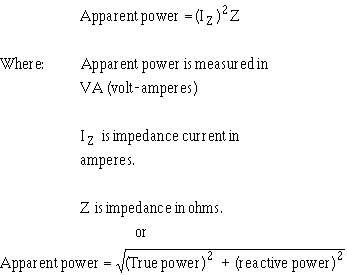voltage source "sees." Apparent power is the combination of true power and reactive power. Apparent power is not found by simply adding true power and reactive power just as impedance is not found by adding resistance and reactance. ">Custom SearchCalculating Apparent Power in AC Circuits. Apparent power is the power that appears to the source because of the circuit impedance. Since the impedance is the total opposition to ac, the apparent power is that power the voltage source "sees." Apparent power is the combination of true power and reactive power. Apparent power is not found by simply adding true power and reactive power just as impedance is not found by adding resistance and reactance. To calculate apparent power, you may use either of the following formulas:For example, find the apparent power for the circuit shown in figure 4-10.Recall that current in a series circuit is the same in all parts of the circuit.Q.25 What is apparent power? Q.26 What is the unit of measurement for apparent power? Q.27 What is the formula for apparent power?Integrated Publishing, Inc. - A (SDVOSB) Service Disabled Veteran Owned Small Business